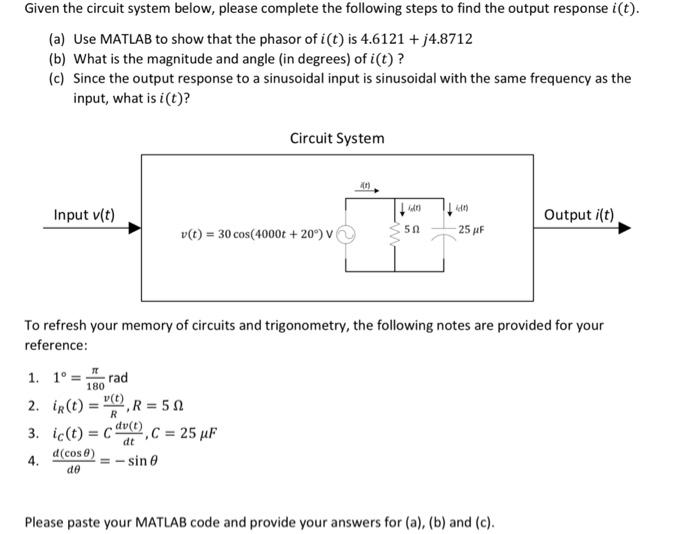# (Solved): Given the circuit system below, please complete the following steps to find the output response i(t ...Given the circuit system below, please complete the following steps to find the output response . (a) Use MATLAB to show that the phasor of is (b) What is the magnitude and angle (in degrees) of ? (c) Since the output response to a sinusoidal input is sinusoidal with the same frequency as the input, what is ? To refresh your memory of circuits and trigonometry, the following notes are provided for your reference: 1. 2. 3. 4. Please paste your MATLAB code and provide your answers for (a), (b) and (c).

We have an Answer from Expert